# HSSlive: Plus One & Plus Two Notes & Solutions for Kerala State Board

## AP Board Class 9 Physical Science Chapter 10 Work and Energy Textbook Solutions PDF: Download Andhra Pradesh Board STD 9th Physical Science Chapter 10 Work and Energy Book AnswersAP Board Class 9 Physical Science Chapter 10 Work and Energy Textbook Solutions PDF: Download Andhra Pradesh Board STD 9th Physical Science Chapter 10 Work and Energy Book Answers

## Andhra Pradesh State Board Class 9th Physical Science Chapter 10 Work and Energy Books Solutions

 Board AP Board Materials Textbook Solutions/Guide Format DOC/PDF Class 9th Subject Maths Chapters Physical Science Chapter 10 Work and Energy Provider Hsslive

2. Click on the Andhra Pradesh Board Class 9th Physical Science Chapter 10 Work and Energy Answers.
3. Look for your Andhra Pradesh Board STD 9th Physical Science Chapter 10 Work and Energy Textbooks PDF.
4. Now download or read the Andhra Pradesh Board Class 9th Physical Science Chapter 10 Work and Energy Textbook Solutions for PDF Free.

## AP Board Class 9th Physical Science Chapter 10 Work and Energy Textbooks Solutions with Answer PDF Download

Find below the list of all AP Board Class 9th Physical Science Chapter 10 Work and Energy Textbook Solutions for PDF’s for you to download and prepare for the upcoming exams:

### 9th Class Physical Science 10th Lesson Work and Energy Textbook Questions and Answers

Question 1.
Define work and write its units. (AS 1)
Work : Work can be defined as the product of the force (F) and the distance (s) moved along the direction of the force.
Work done = Force × displacement = F × s
[This formula is used in only translatory motion of the object]

1. Work is a scalar quantity.
2. Unit of work is ‘N – m’ or ‘Joule’ (J).

Question 2.
Give few examples where displacement of an object is in the direction opposite to the force acting on the object. (AS 1)

• When a ball is thrown up, the motion is in upward direction, whereas the force due to earth’s gravity is in downward direction.
• If a ball is moving on plain ground, will get stopped after sometime, due to frictional force acting on it in opposite direction.

Question 3.
Identify the wrong statement among the following. Rewrite them by making necessary corrections. (AS 1)
a) Work and energy have different units.
Correction :
Work and energy have same units.

b) When an aeroplane takes off, the work done by its weight is positive.
Answr:
Correction : When an aeroplane takes off, the work done by its weight is negative.

c) The potential energy of spring increases when it is extended and decreases when it is compressed.
Correct.

d) If the work done by external forces on a system is negative then the energy of the system decreases.
Correct.

e) When a body is falling freely from a height, its kinetic energy remains constant.
Correction : When a body is falling freely from a height, its K.E. increases and P.E. decreases, but the total energy of the body remains constant.

f) The unit of power is watt.
Correct.

Question 4.
What is mechanical energy? (AS 1)
The sum of the kinetic energy and the potential energy of an object is called its mechanical energy.

Question 5.
State the principle of conservation of energy. (AS 1)
Energy can neither be created nor destroyed. It can only be changed from one form to another.

Question 6.
When you lift a box from the floor and put it on an almirah the potential energy of the box increases but there is no change in its kinetic energy. Is it violation of conservation of energy? Explain. (AS 7)

• When the box is on the floor, it has no K.E., but has some P.E.
• Its P.E. increases as it is lifted and put on an almirah.
• In this event, the energy produced by the person converts into K.E. and the total M.E. remains same.
• Hence it is not the violation of conservation of energy.

Question 7.
One person says that potential energy of a particular book kept in an almirah is 20 J and other says it is 30 J. Is one of them necessarily wrong? Give reasons. (AS 2, AS 1)
Two cases will arise in this situation.
Case – I :
One of them will be wrong, if both of them kept the book in the same shelf of the almirah.

Case – II :
Both of them will be correct, if the first person keeps the book in lower shelf and the second one keeps the book in upper shelf of the almirah.

Question 8.
In which of the following cases is the work done positive or zero or negative? (AS 1)
a) Work done by the porter on a suitcase in lifting it from the platform on to his head.
Positive

b) Work done by the force of gravity on suitcase as the suitcase falls from porter’s head.
Positive

c) Work done by the porter standing on platform with suitcase on his head.
Zero

d) Work done by force of gravity on a ball thrown up vertically up into the sky.
Negative

e) Work done by force applied by hands of a man swimming in a pond.
Negative

Question 9.
What is potential energy? Derive an equation for gravitational potential energy of a body of mass ‘m’ at a height ‘h’. (AS 1)
Potential energy:
The energy possessed by an object because of its position or shape is called its potential energy.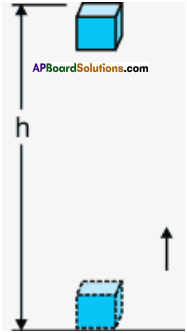Potential energy of a body at height (Or) Gravitational potential energy :

1. The gravitational potential energy of an object at a point above the ground is defined as the work done in raising it from the ground to that point against gravity.
2. Consider an object of mass ‘m’ raised to height ’h’ from the ground.
3. A force is required to do this.
4. The minimum force required to raise the object is equal to the weight of the object (mg).
5. The object gains energy equal to the work done on it.
6. Let the work done on the object against gravity be ‘W’.
∴ W = Force × displacement
= mg × h
W = mgh
7. The object gains the energy equal to the mgh’ units.
8. This energy is the potential energy of the object at a height ‘h’.
∴ P.E. = mgh

Question 10.
When an apple falls from a tree what happens to its gravitational potential energy just as it reaches the ground? After it strikes the ground? (AS 7)

• Let us imagine an apple of mass ‘m’ falls from a height h.
• Before starting to fall down, its velocity is zero. Hence its energy is purely potential.
• When it falls down, its gravitational potential energy gradually converts into kinetic energy, but the entire energy in the system remains same.
• When it strikes the ground, its energy is fully kinetic, till its velocity becomes zero.

Question 11.
Let us assume that you have lifted a suitcase and kept it on a table. On which of the following does the work done by you depend or not depend? Why?
a) The path taken by the suitcase
b) The time taken by you in doing so
c) The weight of the suitcase
We know that P.E. = mgh
a) The work done by you does not depend on the path taken by the suitcase, because the height from the ground to the top of the table is fixed in this case, (h constant)

b) The work done by you does not depend the time taken, in doing so, because the work done is same. If you take less time your power will be more otherwise the power is less.

c) The work done by you depends on the weight of the suitcase. As the weight of the suitcase increases, the work done will also increase (See the formula P.E. = mgh).

d) The work done by you depends on your weight, we know that a person with more weight can Mft the suitcase easily.

Question 12.
When you push your bicycle up an incline, the potential energy of the bicycle and yourself increase. Where does this energy come from? (AS 7)
The energy inside our body causes increase in the P.E. of the bicycle and ourself.

As we are pushing the bicycle upon incline, the work done by the gravitational force is negative. Hence the energy loses.

Question 13.
Why does a person standing for a long time get tired when he does not appear to be doing any work? (AS 7)
1) A person standing for a long time, does not appear to be doing any work, because there is no displacement.
2) Eventhough work has not been done, the person gets tired.
3) This is due to the utilization of energy produced in his body.

Question 14.
What is kinetic energy? Derive an expression for the kinetic energy of a body of mass ‘m’ moving at a speed ‘v’. (AS 1)
Kinetic energy :
The energy possessed by an object due to its motion is called kinetic energy.

Numerical expression for K.E.:
1) Let us assume that an object of mass (m) is at rest on a smooth horizontal plane as shown in figure.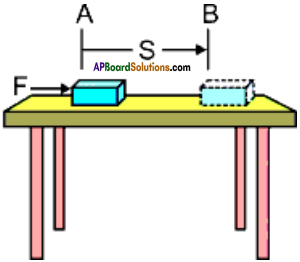2) Let be displaced through a distence ‘s’ from the point A to B by a force (F) acting upon it in the direction of the displacement.
3) In the horizontal direction the net force ‘Fnet‘ is equal to the force applied ‘F’.
∴ W = Fnet.s = F.s …………. (1)
4) Let the work done on the object cause a change in its velocity from ‘u’ to ‘v’ anr the ‘acceleration produced be ‘a’.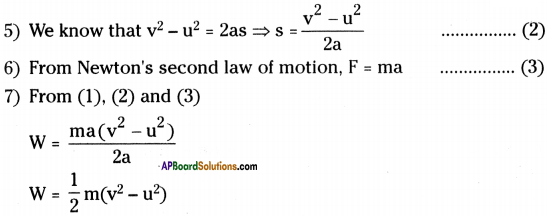8) As we Have assumed that object is at rest, then the initial velocity u = 0.
∴ W = 12 mv²

9) We know that K.E. of a body moving with certain velocity is equal to work done on the object to acquire that velocity from rest.

10) Thus the K.E. of an object of mass’m’ and moving with velocity V is equal to
K.E = 12 mv²

Question 15.
A free-fall object eventually stops on reaching the ground. What happens to its kinetic energy? (AS 1)

• Its kinetic energy becomes ‘zero’.
• As the ball stops on reaching the ground, its final velocity ‘v’ becomes zero.
• Hence the K.E. = 12 mv² = 12.m.0 = 0

Question 16.
A man carrying a bag of total mass 25 kg climbs up to a height of 10 m in 50 seconds. Calculate the power delivered by him on the bag. (AS 1)
Mass of the bag = 25 kg ; Height ‘h’ = 10 m
Acceleration due to gravity g = 9.8 m/s²
The P.E. of the man = mgh = 25 × 9.8 × 10 = 2450 J
The work done by the man is equal to the P.E. possessed by the man.
∴ The work done by the man (W) = 2450 J ; Time (t) = 50 sec.
∴ Power delivered by the man on the bag = Wt=2450 J50sec = 49 watts

Question 17.
A 10 kg ball is dropped from a height of 10 m. Find (a) the initial potential energy of the ball, (b) the kinetic energy just before it reaches the ground and (c) the speed just before it reaches the ground.
Mass of the ball = 10 kg ; It is dropped from a height h = 10 m
Acceleration due to gravity g = 9.8 m/sec²
a) The initial P.E. of the ball = mgh = 10 × 9.8 × 10 = 980 J
b) We know that the energy is conserved in a freely falling object at anywhere in its journey.
∴ The K.E. just before it reaches the ground = 980 J

c) K.E. just before it reaches the ground = 980 J ;
Speed = v m/s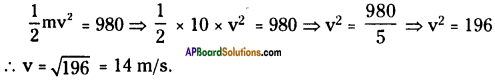Question 18.
Calculate the work done by a person in lifting a load of 20 kg from the ground and placing it 1 m high on a table. (AS 1)
Mass of a load = 20 kg ; Height h = 1 m
Acceleration due to gravity = 9.8 m/s²
The work done by a person in lifting the load is equal to the P.E.
P.E. = mgh = 20 × 9.8 × 1 = 196 J

Question 19.
Find the mass of a body which has 5 J of kinetic energy while moving at a speed of 2 m/s. (AS 1)
KE = 5 J
Speed v = 2 m/sec
Mass of the body = m kg.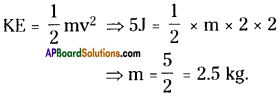Question 20.
A cycle together with its rider weighs 100 kg. How much work is needed to set it moving at 3 m/s?
Mass of the bicycle with its rider = 100 kg ; Speed = 3 m/s Amount of work needed to set it moving is equal to its K.E.
K.E. = 12mv² = 12 × 100 × 3 × 3 = 450 J
∴ 450 J work is needed.

Question 21.
When the speed of a ball is doubled its kinetic energy (AS 1)
A) remains same
B) gets doubled
C) becomes half
D) becomes 4 times
D) becomes 4 time

Question 22.
Two bodies of unequal masses are dropped from the top of a building. Which of the following is equal for both bodies at any instant?
A) Speed
B) Force of gravity
C) Potential energy
D) Kinetic energy
B) Force of gravity

Question 23.
A man with a box on his head is climbing up a ladder. The work done by the man on the box is ……….
A) Positive
B) Negative
C) Zero
D) Undefined
A) Positive

Question 24.
A porter with a suitcase on his head is climbing up steps with uniform speed. The work done by the “weight of the suitcase” on the suitcase is ……
A) Positive
B) Negative
C) Zero
D) Undefined
B) Negative

Question 25.
How will the increasing energy needs and conservation of energy influence international peace, cooperation and security? Discuss.
1) The increasing energy needs and conservation of energy influence international peace, co-operation and security.

2) For example, many great nations depend on the gulf countries and many countries for fuel (Petrol, diesel, etc.). The need of fuel brings co-operation among the countries. Also the need of energy (electricity, fuel, etc.) link all nations to a small circle so that there comes the peace, co-operation and security.

3) Atomic energy, thermal energy, chemical energy, etc. bring unity among all nations.

Question 26.
How would you assess the role of energy conversion occurring naturally in maintaining ecological balance of nature?

• The chemical changes in the sun is providing us the heat energy and light energy to the earth.
• The heat energy of the sun evaporates the water into water vapour. This helps us to get heavy rainfall.
• The solar energy is taken by the plant to change CO2, water and chloroplast into starch which is the food of the plant. This process is called photosvnthesis.
• In the photosynthesis process the plant releases O2 (oxygen).
• The leguminous (Pea plants) plants help the bacteria present at their roots. In return they help the plants to fix nitrogen in them The nitrogen cycle is maintained.
• From the above examples we can understand that the energy conversion is helpful in maintaining ecological balance of nature.

Question 27.
Collect pictures showing various situation where potential energy possessed by an object depends on its shape and position. Prepare a scrap book.
Note : Students can collect pictures of different objects at different heights, with different shapes.
e.g.: 1) A stone on a hill.
2) A book on a table and a pen on a table, etc.
Paste these pictures in your scrap book.

Question 28.
Draw a diagram to show conservation of mechanical energy in case of free falling body.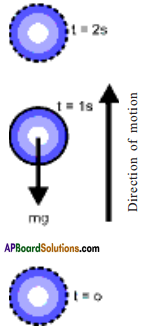### 9th Class Physical Science 10th Lesson Work and Energy InText Questions and Answers

9th Class Physical Science Textbook Page No. 161

Question 1.
How are these works being done?
All the works mentioned above are being done by utilizing human muscular energy or electrical energy.

Question 2.
What do you need to do these works?
Both human beings or machines need energy to do work.

Question 3.
Where does the energy spent go ultimately?
The energy spent will be converted into another form to complete the task.

Question 4.
Is there any transfer of energy while work is being done?
Yes. We can observe the transfer of energy while work is being done. For example, in fan, electrical energy is converted into mechanical energy. In heater, electrical energy is converted into heat energy, etc.

Question 5.
Can we do any work without transfer of energy?
No, we cannot do any work without transfer of energy.

9th Class Physical Science Textbook Page No. 162

Question 6.
What is work?
When a force is applied on an object and a displacement is observed, the work is said to be done.

Question 7.
Why is there difference between general view of work and scientific view of work?
In general view, work is said to be done while reading, writing, eating or even standing for some time. We cannot observe any displacement in these cases. But in scientific view, work is said to be done when there is a displacement in the direction of force applied.

9th Class Physical Science Textbook Page No. 163

Question 8.
Observe the following examples.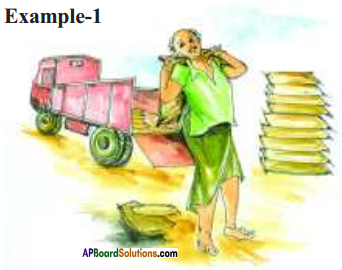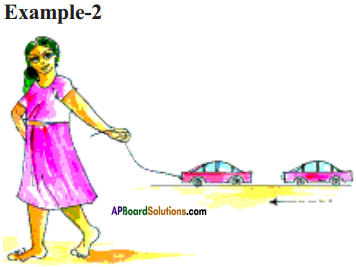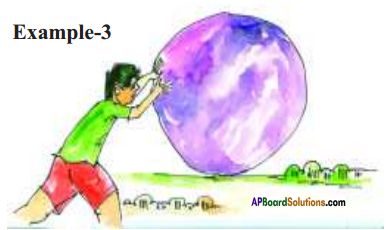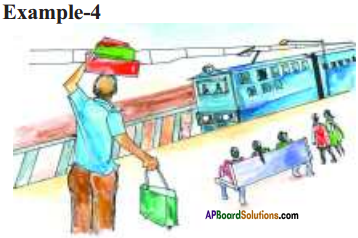a) Are all the people mentioned in the above examples doing work ?
No.
In example (1) and (2) there is displacement. Hence they are doing work.
In example (3) and (4) there is no displacement. Hence they are not doing any work.

b) How do you define work?
Work is defined as the product of force and displacement.
W = F × s

9th Class Physical Science Textbook Page No. 165

Question 9.
What would be the work done when the force on the object is zero?
We know work done W = F × s
Here F = 0
⇒ W = 0 × s = 0
∴ The work done is zero.

Question 10.
What would be the work done when the displacement of the object is zero?
Work done W = F × s
Here s = 0
⇒ W = F × 0 = 0
∴ The work done is zero.

Question 11.
Can you give some examples, where the displacement of the object is zero?

• When a boy pushes a wall, the displacement in the wall is zero.
• When a boy stands for some time at one place, then there is no displacement in the boy.
• Wind causes the swing in trees, but the tree does not have any displacement.

9th Class Physical Science Textbook Page No. 166

Question 12.
What happens to the speed of the ball while it moves up?
The speed of the ball while it moves up decreases gradually, due to negative gravitational force acting on it.

Question 13.
What is the speed at its maximum height?
The speed of the ball at its maximum height is zero.

Question 14.
What happens to the speed of the ball during its downward motion?
During its downward motion, the ball starts from zero velocity*and its velocity gradually increases due to the positive gravitational force acting on it.

9th Class Physical Science Textbook Page No. 167

Question 15.
What is energy?
Energy is the capability to do work.

Question 16.
How can we decide that an object possess energy or not?
The capacity of doing work by an object on another object depends on position and state of the object which is doing work.

Question 17.
What will happen to the plate? Why?
The plate breaks into pieces, because of the potential energy of the metal ball.

Question 18.
What changes do you notice? Why?
The toy car is at rest before winding the key but the same toy gets energy to move when the key attached to it is wound up.

9th Class Physical Science Textbook Page No. 168

Question 19.
What could be the reason?

• The reason for breaking up of the plate is the potential energy of the metal ball.
• The reason for moving the toy car is the potential energy of the spring in the toy.

Question 20.
Where does this energy go?
The energy is transferred from one object to another.

Question 21.
Is there any energy transfer between the object doing the work and the object on which work has been done?
Yes. In case of the toy car, the potential energy of the wounded spring is transferred to car and made the toy car to move.

Question 22.
Can any force do work without energy transfer?
No.

9th Class Physical Science Textbook Page No. 169

Question 23.
Where do we get energy from?
The Sun is the biggest natural and primary source of energy for us. Many other secondary sources are derived from the sun. We can also get energy from the interior of the earth and from tides of the sea.

Question 24.
Can you think of other sources of energy?
The other natural sources of energy are wind energy, tidal energy, gravitational energy, etc. The artificial sources of energy are electrical energy, heat energy, muscular energy, etc.

Question 25.
Are there sources of energy which are not dependent on the Sun?
Energy can exists in several forms like mechanical energy, light energy, thermal energy, sound energy, electrical energy, magnetic energy, etc. These energies, though they are artificial, they ultimately depend on the Sun.

Question 26.
Do you know why a person gets tired standing at a place for long time?
Though the person standing is not doing any work externally, a lot of work is being done inside the body.

The muscles of the body become stretched when he stands for long time and heart has to pump more blood to muscles. This leads to loss of energy inside the body and hence he gets tired.

9th Class Physical Science Textbook Page No. 176

Question 27.
How do green plants produce food?
Green plants produce food from sunlight, by means of photosynthesis.

Question 28.
How are fuels like coal and petroleum formed?
Dead plants hurried deep below the earth’s surface for millions of years get converted to fuels like petroleum and coal which have chemical energy stored in them.

Question 29.
What kind of energy conversions sustain the water cycle in nature?
Water cycle and conversation of energies :
1) The Sun heats water in oceans and seas. Then water evaporates as water vapour into the air.
Here potential energy converts into kinetic energy.

2) Rising air currents take the vapour up into the atmosphere. There cooler temperatures cause it to condense into clouds.
Here kinetic energy converts into potential energy.

3) Air currents move water vapour around the globe, cloud particles collide, grow and fall out as rain.
Here potential energy converts into kinetic energy.

4) Run off and ground water are stored as fresh water in lakes. Some precipitation falls as snow or hail.
Here kinetic energy converts into potential energy.

9th Class Physical Science Textbook Page No. 178

Question 30.
Do all of us do the work at the same rate?
The rate of work done by all of us will not same, because the capacity to do work differs from person to person depending on the weight, nature of work, etc.

Question 31.
Is the energy spent by the force doing work the same every time?
No. The energy spent by the force doing work depends on the capacity of the machine or the capacity of a person.

Question 32.
Do the machines consume or transfer energy at same rate every time while doing a particular work?
No. For example, consider two grinders of different wattages. The grinder with high wattage can perform fast. In this the energy transfer is faster. The grinder with less wattage can perform slowly. In this the energy transfer is slow.

9th Class Physical Science Textbook Page No. 179

Question 33.
Whose argument is correct?
Answr:
Raheem’s argument is correct, in view of work done.
Second labourer’s argument is correct in view of working hours.

Question 34.
Is work done in two cases same?
The work done in two cases is same.

Question 35.
Why is there a change in rate of doing work?
The rate of doing work is different, as the two labourers completed in different times.

9th Class Physical Science Textbook Page No. 165

Question 37.
A wooden chair is dragged on the level floor and brought to the same place. Let the distance covered be ‘s’ and frictional force acted on the chair by the floor be T. What is the work done by the frictional force?
The displacement of the chair is zero. Hence the work done is zero.

9th Class Physical Science Textbook Page No. 166

Question 38.
Lift an object up from the ground. Work done by the force exerted by you on the object moves it in upward direction. Thus the force applied is in the direction of displacement. However there exists a force of gravitation on the object at the same time
• Which one of these forces is doing positive work?
• Which one is doing negative work?
• Give reasons.

• Force applied by us on the object, because the object moves in the direction of force applied.
• Force of gravitation on the object is doing negative work. Here the object is moving against to gravitational force.

9th Class Physical Science Textbook Page No. 169

Question 39.
What would happen if nature does not allow the transfer of energy? Discuss with few examples.
If nature could not allow the transfer of energy, the normal life is not possible, e.g.:
1) If the solar energy is not transferred into chemical energy, in which plants prepare their food, we cannot find any plant on the earth. Life without plants is impossible. We can not get food, cloth, shelter and fresh air for our survival.

2) Water cycle is also an example of transfer of energy in nature. If this does not take place, ground water cannot be recharged, we cannot find any water further.

9th Class Physical Science Textbook Page No. 172

Question 40.
a) Why is it easier to stop a lightly loaded truck than heavier one that has equal speed?
Due to its light weight, the truck with lighter load is easy to stop.

b) Does the kinetic energy of a car change more when it goes from 10 m/s to 20 m/s or when it goes from 20 m/s to 30 m/s?
Case – I :
K.E.(I) = 12 × m × 10 × 10 = 50m J
K.E.(F) = 12 × m × 20 × 20 = 200m J
Difference in K.E. = 200m – 50m = 150m J

Case – II :
K.E.(I) = 12 × m × 20 × 20 = 200m J
K.E.(F) = 12 × m × 30 × 30 = 450m J
Difference in K.E. = 450m – 200m = 250m J

c) A person starts from rest and begins to run. The runner puts a certain momentum into himself. What is the momentum of ground? And the runner puts a certain amount of kinetic energy into himself. What is the kinetic energy of the ground?
Here two cases arise.
Case – I :
In view of the observer, ground has no velocity. Hence the momentum and kinetic energy of the ground will be zero.

Case – II :
In view of the runner, ground has a velocity equal to the velocity of the runner.

1. The momentum of ground is equal to the momentum of the runner in magnitude but opposite in direction.
2. The K.E. of ground is also equal to the K.E of the runner in magnitude but opposite in direction.

9th Class Physical Science Textbook Page No. 175

Question 41.
Does the international space station have gravitational potential energy?
International space station is situated in space where the gravitational force is zero. Hence the gravitational potential energy is zero.

9th Class Physical Science Textbook Page No. 178

Question 42.
a) Someone wanting to sell you a super ball claims’ that it will bounce to a height greater than the height from which it is dropped. Would you buy this ball? If yes explain, if not explain.
Yes. I will buy this ball. When the applied force is more, the ball bounce to a height greater than the hefght from which it is dropped.

b) A ball, initially at the top of the inclined hill, is allowed to roll down: At the bottom its speed is 4 m/s. Next, the ball is again rolled down the hill, but this time it does not start from rest. It has an initial speed of 3 m/s. How fast is it going when it gets to the bottom?
A ball start from the top of an inclined plane u} = 0 m/s; v, = 4 m/s.
Let the acceleration a’ and distance ’s’.
v1² – u1² = 2as …………. (1)
Now the ball is rolled down from the top of an inclined plane with a velocity 3 m/s.
∴ u2 = 3 m/s; v2 =?
Here the acceleration of the inclined plane is ‘a’ and the distance is ‘s’.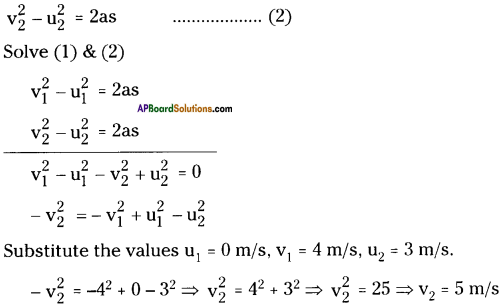9th Class Physical Science Textbook Page No. 179

Question 43.
The work done by a force F1 is larger than the work done by another force F2. Is it necessary that power delivered by F1 is also larger than that of F2? Why?
Power delivered by F1 is may or may not be larger than F2.
Two cases arise.
Case – I :
If the displacement is same, as F1 > F2, the power delivered by F1 is larger than F2.

Case – II :
If the displacement is different, power delivered by F1 may not be larger than F2.

9th Class Physical Science 10th Lesson Work and Energy Activities

Activity – 1

Question 1.
Read Ex : 1, 2, 3, and 4 from page 162 and 163. Now fill the following table.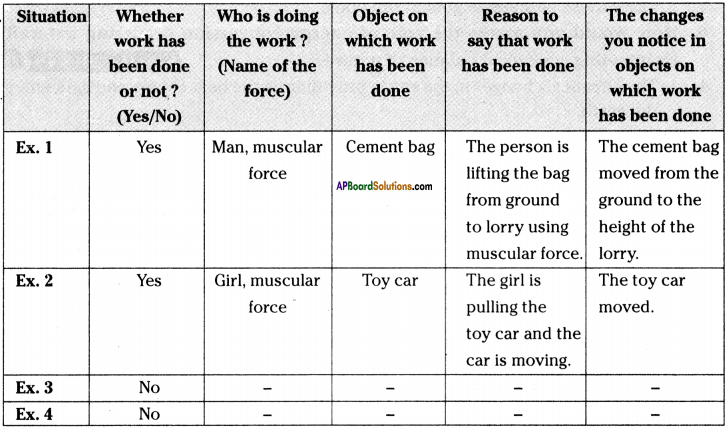Activity – 2

Question 2.
Explain the relation between work done on an object and energy of the object with an activity.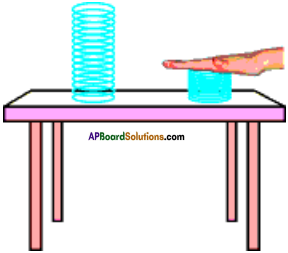1. Take a hard spring and keep it on the table as shown in the figure.
2. Now compress the spring with your palm and release it after few seconds.
3. We will notice that when a spring is being compressed there is a change in its size.
4. When it is released it gains some energy and may even jump from the table.
5. The work done by your palm on the spring increases its energy and makes it to jump.
6. Thus we can conclude that the object which does work loses energy and the object on which work has been done gains energy.

Activity – 3

Question 3.
List the energy sources.
The main source of energy is the sun. Many other secondary sources of energy are electrical energy, magnetic energy, chemical energy, muscular energy, geothermal energy, energy from fossil fuels, etc.

Activity – 4

Question 4.
Explain the energy of moving objects with an activity.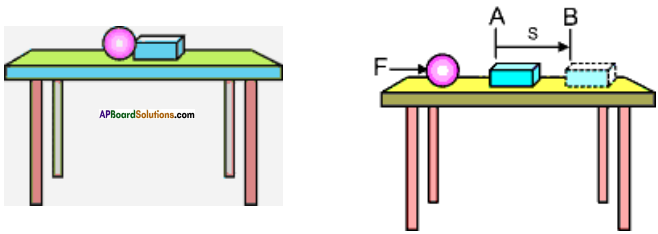1. Consider a metal ball and a hollow plastic block which are kept on a table side by side.
2. Now, suppose that the ball is separated from the block and brought to one end of the table and pushed to roll on the table with speed ‘v’.
3. We may notice that, the ball hits the plastic block and displaces it from point A to B.
4. Thus a moving ball is more energetic than the ball at rest.
5. We can conclude that a body possesses more energy when it is moving than when it is at rest.

Activity – 5

Question 5.
Explain potential energy with the help of a bow.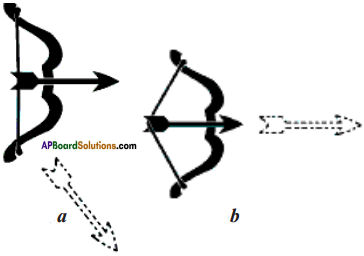1. Take a bamboo stick and make a bow as shown in figure.
2. Place an arrow made of a light stick on it with one end supported by the string of the bow and stretch the string gently and release the arrow.
3. We notice that the arrow gets separated from the bow and falls down on the ground.
4. Now place the arrow on the bow with one end supported by the string, applying more force and release the arrow.
5. We observe that the arrow flies with great speed into the air.
6. From this we can conclude that the bow in normal shape is not able to push the arrow, but when we stretch the string, it acquires energy to throw the arrow into air with great speed.
7. The energy acquired by the bow due to change in its shape is known as its potential energy.

Activity – 6

Question 6.
Write an activity to show the energy in stretched rubber band.
Take a rubber band hold it at one end and pull it from the other end. Then release the rubber band at one of the ends.

When we release the rubber band, it strikes our hand with great force. This force is due to the energy acquired by the rubber band by stretching it.

Activity – 7

Question 7.
Show that the object at some height possesses energy.

1. Take a heavy ball.
2. Drop it on a thick bed of wet sand from different heights from 25 cm to 1.5 m.
3. Observe the depression created by the ball on die bed of sand. Compare the depths of these depressions.
4. When the ball is dropped from different heights, the depressions created by the ball on the bed of sand will be different. ‘
5. As the height from which the ball was dropped increases, the depth of depression also increases.
6. Thus we can conclude that a body at some height possesses energy.

Activity – 8

Question 8.
List out the energy conversions in nature and in day to day life.
Discuss various ways of energy conversions in nature as well as in our day to day activities and make a separate list of situations for natural conversions of energy and energy conversions in day to day life and write them in the given tables.
Table

 Sl.No. Situation of energy conversion in nature 1 Heat energy from the sun used for preparing food by plants gets con­verted into chemical energy. 2 Heat energy of the earth’s crust is useful in the formation of fossil fuels like petroleum, coal, etc. 3 Heat energy from the sun is converted into wind energy and wave energy. 4 Food we take will convert into energy. 5 Water cycleHeat energy → Water vapour → water (rain)

Table

 Situation of energy conversion Gadgets / appliances used for energy conversion 1. Conversion of electrical energy into mechanical energy Electric fan 2. Conversion of mechanical energy into electrical energy Dynamo 3. Conversion of chemical energy into electrical energy Cell 4. Conversion of chemical energy into mechanical energy Motor vehicles 5. Electrical energy into heat energy Electric stove 6. Electrical energy into light energy Bulb 7. Solar energy into electrical energy Solar lamp 8. Wind energy to electrical energy Wind mills 9. Gravitational potential energy to electrical energy Hydro electric power

Activity – 9

Question 9.
Write an activity which shows the conservation of mechanical energy.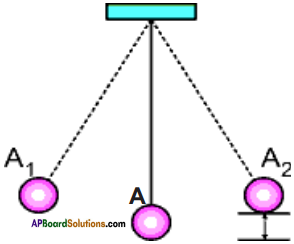1. Take a long thread say 50-60 cm long.
2. Attach a small heavy object like a metal ball at one end.
3. Tie other end to a nail fixed to the wall as shown in figure.
4. Now pull the bob of the pendulum to one side to the position A1 and release it.
5. The bob swings towards opposite side and reaches the point A2. It repeats the motion over and over again.
6. The potential energy of the bob is minimum at A and reaches maximum at A1 because the height of the bob is maximum at that position.
7. When the bob is released from this point (A1), its P.E. decreases and K.E. starts increasing slowly.
8. When the bob reaches the position A, its K.E. reaches maximum, and P.E. becomes minimum.
9. As the bob proceeds from A to A2, its P.E. increases slowly and becomes maximum at A2.
10. The total P.E. and K.E. at any point on the path of motion during the oscillation of the pendulum.
11. Thus the total mechanical energy in the system of pendulum remains constant.

Activity – 10

Question 10.
Calculate the total energy of free-fall at different heights.
An object of mass 20 kg is dropped from a height of 4 m. Compute the potential and kinetic energy in each case and write the values in the table. (Take g = 10 ms-2)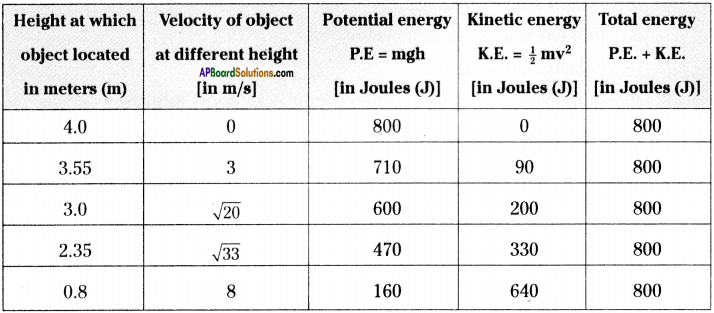a) What do you say about total energy of system of freely falling body?
In a freely falling body, the total energy of system (i.e. the sum of potential energy and kinetic energy) is same at any instance of its travel.

b) Is the mechanical energy conserved in the system?
The sum of potential energy and kinetic energy at any instance of its travel is same. Hence we can say that the mechanical energy is conserved in the system.

## Andhra Pradesh Board Class 9th Physical Science Chapter 10 Work and Energy Textbooks for Exam Preparations

Andhra Pradesh Board Class 9th Physical Science Chapter 10 Work and Energy Textbook Solutions can be of great help in your Andhra Pradesh Board Class 9th Physical Science Chapter 10 Work and Energy exam preparation. The AP Board STD 9th Physical Science Chapter 10 Work and Energy Textbooks study material, used with the English medium textbooks, can help you complete the entire Class 9th Physical Science Chapter 10 Work and Energy Books State Board syllabus with maximum efficiency.

## FAQs Regarding Andhra Pradesh Board Class 9th Physical Science Chapter 10 Work and Energy Textbook Solutions

#### Can we get a Andhra Pradesh State Board Book PDF for all Classes?

Yes you can get Andhra Pradesh Board Text Book PDF for all classes using the links provided in the above article.

## Important Terms

Andhra Pradesh Board Class 9th Physical Science Chapter 10 Work and Energy, AP Board Class 9th Physical Science Chapter 10 Work and Energy Textbooks, Andhra Pradesh State Board Class 9th Physical Science Chapter 10 Work and Energy, Andhra Pradesh State Board Class 9th Physical Science Chapter 10 Work and Energy Textbook solutions, AP Board Class 9th Physical Science Chapter 10 Work and Energy Textbooks Solutions, Andhra Pradesh Board STD 9th Physical Science Chapter 10 Work and Energy, AP Board STD 9th Physical Science Chapter 10 Work and Energy Textbooks, Andhra Pradesh State Board STD 9th Physical Science Chapter 10 Work and Energy, Andhra Pradesh State Board STD 9th Physical Science Chapter 10 Work and Energy Textbook solutions, AP Board STD 9th Physical Science Chapter 10 Work and Energy Textbooks Solutions,
Share: